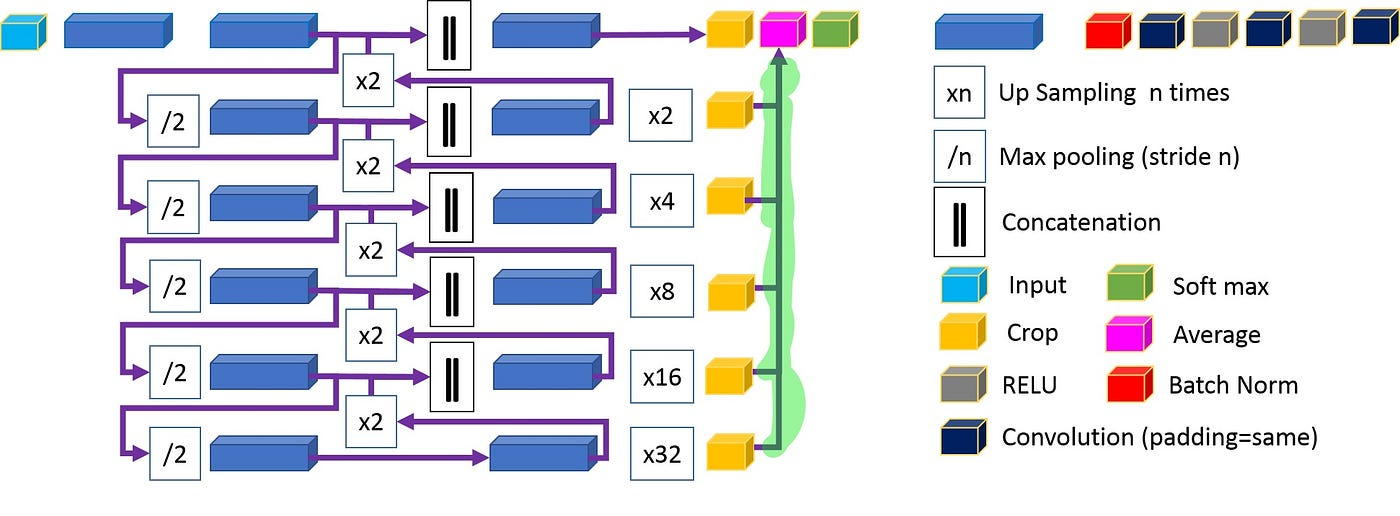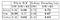# Yet Another Imbalance Data Handling Approach

This is a short review for a recent paper , accepted in MICCAI2018, doing medical image semantic segmentation. The paper employs a modified U-Net architecture to segment kidney vessels. To avoid medical terminology, I substitute foreground kidney vessels like artery with F1, vein with F2 and ureter with F3. Thus, the semantic segmentation problem has a background class B and three foreground classes (F1, F2, F3).

The paper adds few residual links to U-Net architecture to avoid vanishing gradient problem. The next figure highlights in green the residual links between the crop and average layers. Residual links help propagate gradient to early layers. Further evaluation for integrating residual links in U-Net architecture is available in .KID-Net architecture. The two contradicting phases are colored in blue. The down-sampling and up-sampling phases detect and localize features respectively. The segmentation result, at different scale levels, are averaged to compute the final segmentation.

This article draws attention to another idea in the paper; how to handle imbalance data? The foreground classes (Kidney vessels) are tiny anatomy. All foreground classes combined represent less than five percent while the background class is more than 95%. This is a typical imbalance data scenario. Well-established imbalance data handling techniques are

• Custom weighting like median frequency weighting
• Bootstrapping
• Custom sampling (oversampling and undersampling)

This paper proposes a variant of custom sampling that seems novel to my humble knowledge. The aforementioned methods treat all background pixels/samples equally, e.g. assign small weight. This paper assigns a high weight to a randomly sampled background subset (B’). This approach reduces false positive and improves the overall system performance. I will present a detailed numerical example for this approach, then explain the intuition behind it.

The next figure compares the typical weighting schema versus the paper’s approach using a toy two classes example. A typical approach assigns high weight W_F=90 to the foreground classes occupying 10% of the data volume and small weight W_B=10 to the background class samples. Contrary, this paper divides the background class into B and B’. B’ is a randomly sampled background subset that has the same volume as the minority foreground classes. Thus, the data distribution becomes 80% B, 10% B’ and 10% F. While the background B samples are assigned small weight W_B=20, the foreground F and background B’ samples are assigned high weight W_B’=W_f=40.

To understand this approach, the next table analyzes the loss function for a typical weighting schema. If the network predictions are correct, the network loss equals zero, i.e. not penalized. But things get interesting in case of a wrong prediction. If a true foreground sample, highly weighted W_F=90, is wrongly classified as a background, the network loss equals 90. Contrary, the network loss equals 10 if a true background sample, weighted W_B=10, is wrongly classified as foreground. Such asymmetric loss distribution inclines the network to classify any confusing pixel/sample as foreground. This reduces the network loss by avoiding the high foreground loss (W_F=90). Unfortunately, this increases false positives.

By introducing the background subset B’, that has the same small volume and high weight as the foreground class, false positives decrease. This tackles the undesired incentive to classify any confusing samples/pixel as foreground. With B’ introduced, the loss function becomes as follows

The network will classify any confusing sample/pixel as B’ or F, not B, to avoid the large penalty. But since both B’ and F have the same volume and weight, the network won’t be biased against any of these classes. According to the paper, this reduces false positives and improve classification accuracy as shown in the next table. This imbalance data handling approach is referred to as ‘random sampling’ (RS)Quantitative evaluation for different training schema in two evaluation re- gions. Dynamic Weighting (DW) plus Random Sampling (RS) achieves the highest accuracies.

Qualitative evaluation using CT-scan images are available in the paper.

• My main negative comment is the lack of extensive evaluation. The proposed approach is evaluated on a single dataset, for a single task, semantic segmentation. While the approach seems intuitive, more experiments are still required

 Kid-Net: Convolution Networks for Kidney Vessels Segmentation from CT-Volumes

U-net: Convolutional networks for biomedical image segmentation

 Segmentation of renal structures for image-guided surgery

I write reviews on computer vision papers. Writing tips are welcomed.

## More from Ahmed Taha

I write reviews on computer vision papers. Writing tips are welcomed.